转自知乎-我见过最通俗易懂的KMP算法详解

2 篇文章 1 订阅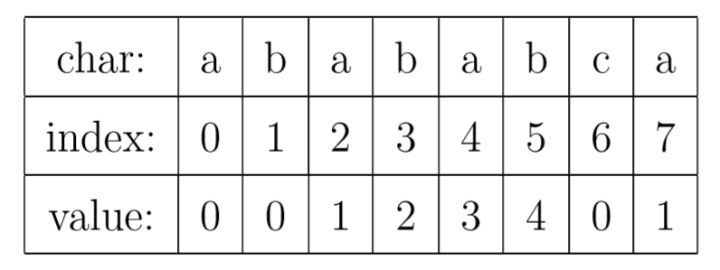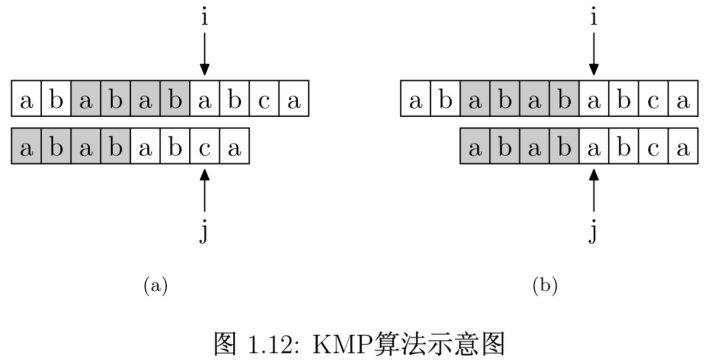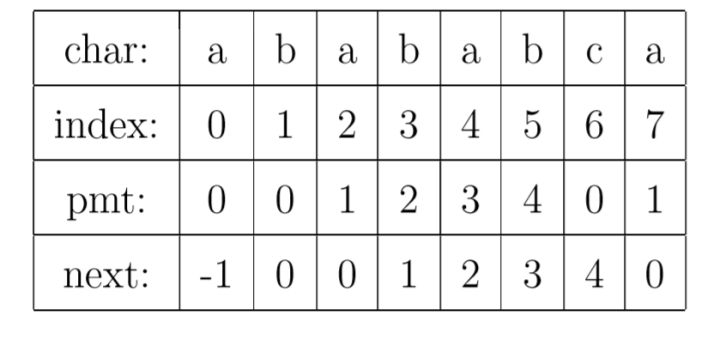int KMP(char * t, char * p)
{
int i = 0;
int j = 0;

while (i < strlen(t) && j < strlen(p))
{
if (j == -1 || t[i] == p[j])
{
i++;
j++;
}
else
j = next[j];
}

if (j == strlen(p))
return i - j;
else
return -1;
}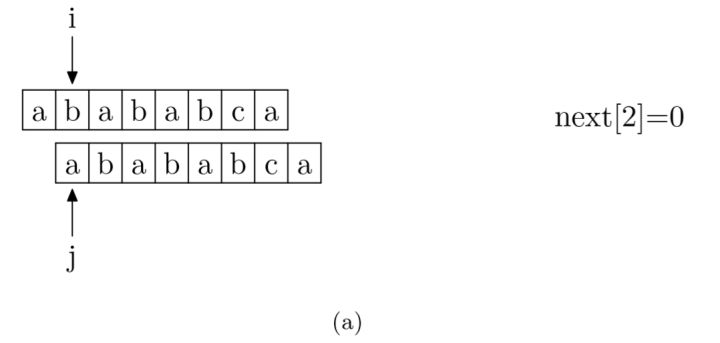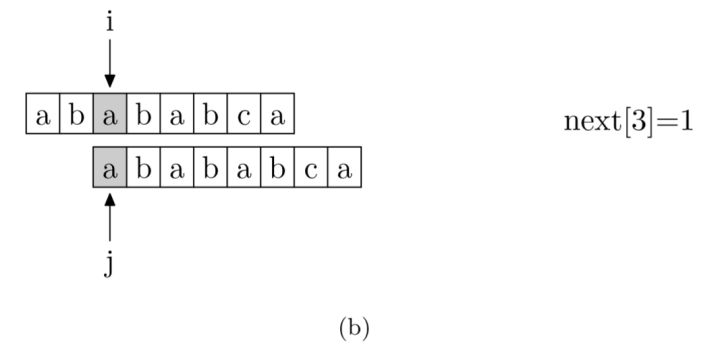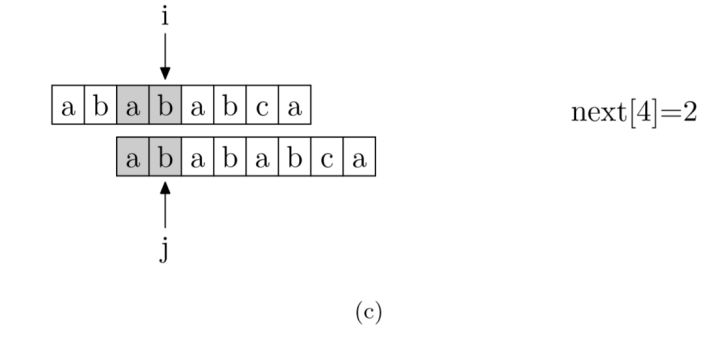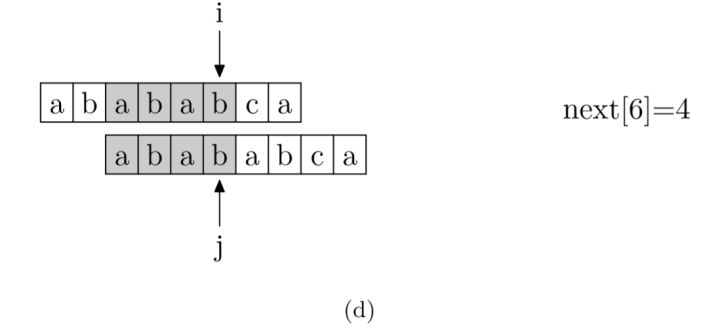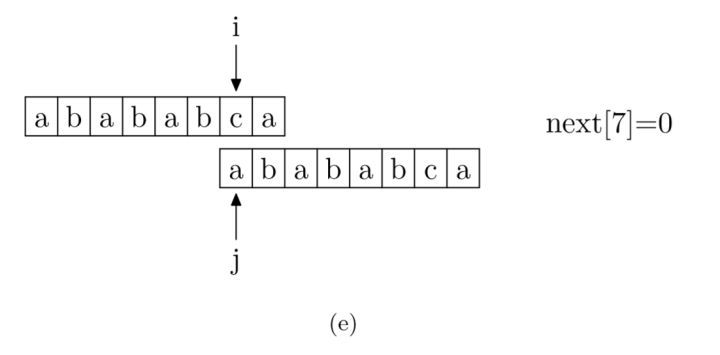void getNext(char * p, int * next)
{
next = -1;
int i = 0, j = -1;

while (i < strlen(p))
{
if (j == -1 || p[i] == p[j])
{
++i;
++j;
next[i] = j;
}
else
j = next[j];
}
}

03-19
01-1632

05-14230
02-28845
02-261327
03-15659
02-0794
04-02109
12-101880
05-03529
12-1072
08-1145
04-2237
08-30295
06-25301
05-02869
05-12277点击重新获取扫码支付余额充值